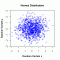I have forgotten

•http://facebook.com/
•https://www.google.com/accounts/o8/id
•https://me.yahoo.com

# Normal

#### CDF

Evaluates the normal cumulative density function.
 double CDF (double x, double m, double s)[inline]

#### PDF

Evaluates the normal probability density function.
 double PDF (double x, double m=0, double s=1)[inline]

#### CDF Inv

Evaluates the inverse of the normal cumulative density function.
 double CDF_inv (double x, double m, double s)[inline]

#### Random Sample

Generates random numbers following a standard normal distribution.
 double sample (double seed=0)